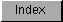```
When you use sampling to produce a waveform, a side effect is caused when
the sampling rate "beats" or combines with the frequency you wish to
produce. This produces two additional frequencies, one at the sampling
rate plus the desired frequency and the other at the sampling rate minus
the desired frequency. This phenomenon is called aliasing distortion.

Aliasing distortion is eliminated when the sampling rate exceeds the
output frequency by at least 7 KHz. This puts the beat frequency outside
the range of the  low-pass filter , cutting off the undesirable
frequencies. Figure 5-5 shows a frequency domain plot of the anti-aliasing
low-pass filter  used in the system.

/|\
|
0db |________ filter
|        \ response
|         \
|          \
|           \      filter passes all frequences below about 5kHz.
|            \
-30db |_____________\_____ ___________________________________________\
|         |         |         |         |         |   /
5kHz     10kHz     15kHz     20kHz     25kHz     30kHz

Figure 5-5: Frequency Domain Plot of Low-Pass Filter

Figure 5-6 shows that it is permissible to use a 12 KHz sampling rate to
produce a 4 KHz waveform. Both of the beat frequencies are outside the
range of the filter, as shown in these calculations:

12 + 4 = 16 KHz
12 - 4 = 8 KHz

/|\  filter            12kHz sampling frequencey
|  response             |
0db |________               |
|        \     Diff.    |      Sum
|       | \     |       |       |
|       |  \    |       |       |
|       |   \   |       |       |
|   4kHz|    \  |       |       |
-30db |_______|_____\_|___ ___|_______|_______________________________\
| |         |         |         |         |         |   /
5kHz     10kHz     15kHz     20kHz     25kHz     30kHz
|
|
desired output frequency

Figure 5-6: Noise-free Output (No Aliasing Distortion)

You can see in Figure 5-7 that is unacceptable to use a 10 KHz sampling
rate to produce a 4 KHz waveform. One of the beat frequencies (10 - 4) is
within the range of the filter, allowing some of that undesirable
frequency to show up in the audio output.

/|\  filter        10kHz sampling frequencey
|  response         |
0db |________           |
|        \ Diff.    |      Sum
|       | \ |       |       |
|       |  \|       |       |
|       |   \       |       |
|   4kHz|   |\      |       |
-30db |_______|___|_\_____|_______|___________________________________\
| |         |         |         |         |         |   /
5kHz     10kHz     15kHz     20kHz     25kHz     30kHz
|
|
desired output frequency

Figure 5-7: Some Aliasing Distortion

All of this gives rise to the following equation, showing that the
sampling frequency must exceed the output frequency by at least 7 KHz, so
that the beat frequency will be above the cutoff range of the
anti-aliasing filter :

Minimum sampling rate = highest frequency component + 7 KHz

The frequency component of the equation is stated as "highest frequency
component" because you may be producing a complex waveform with multiple
frequency elements, rather than a pure sine wave.

```